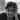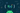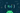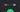Navigate back to the homepage

# Functional Programming in Kotlin - f(2)Hari Vignesh Jayapalan
May 3rd, 2020 · 3 min read## Functions in Kotlin :: Pure Functions

This is the second part of the functional programming series. If you have missed the previous one, start here.

### Series pit stops

In this pit stop, we’ll take a brief look at how we write or represent a function in Kotlin. As a language what functional operations does Kotlin provide us out of the box? We’ll also see about pure functions.

### Pre-requisites

• Basic programming knowledge
• School mathematics
• Previous pit stop

### Functions in Kotlin

As I presume that you already know that in Kotlin we define the function using the fun keyword. I also guess you have played the Kotlin drinking game - drink a shot each time when someone says fun in a Kotlin talk 😛

Jokes apart, Let’s take a look at some of the common functional operations on lists that Kotlin provides out of the box which we may have used in our everyday dev chores without actually realising or comparing it with functional paradigms.

Some of you may know the following functions, but the idea is to provide a different perspective on them when represented mathematically and to digest the functional concepts that we absorbed from the previous pit stop.

#### Dissecting map()

We would have used map frequently on lists in Kotlin. Usually mapping the list of items we receive from an API call to the view data. Let’s connect the dots with the knowledge we consumed from our previous pit stop.

Representation of map

`.css-1chxjt6{position:absolute;right:22px;top:24px;padding:8px 12px 7px;border-radius:5px;color:#6f7177;-webkit-transition:background 0.3s ease;transition:background 0.3s ease;}.css-1chxjt6:hover{background:rgba(255,255,255,0.07);}.css-1chxjt6[data-a11y="true"]:focus::after{content:"";position:absolute;left:-2%;top:-2%;width:104%;height:104%;border:2px solid var(--theme-ui-colors-accent,#6166DC);border-radius:5px;background:rgba(255,255,255,0.01);}@media (max-width:45.9375em){.css-1chxjt6{display:none;}}1map(f: (A) -> B): List<B>`

A functional instance, that takes in a function as an argument and applies to all the elements present in the list of A and provides us with the list of B

`1Mathematically:23        let set of A = { 1, 2, 3, 4, 5, 6 }4        given f(x) = x + 156        map(f: (A) -> B) gives,78        f1: (1) -> 1 + 1 = 29        f2: (2) -> 2 + 1 = 310        ...1112Programatically:1314        val listA = listOf(1, 2, 3, 4, 5, 6)1516        val listB = listA.map { x -> x + 1 } //print1718        // output = [2, 3, 4, 5, 6, 7]`

Fun Fact: When we say map takes a function as an argument, it’s actual representation is map({ x -> x + 1}). But in Kotlin, if the last argument is a function, we can remove the enclosing function brackets. That’s why we have map { x -> x + 1 }

#### Dissecting groupBy()

When we need to group or arrange our lists or mapped to one item, we use groupBy Representation of groupBy

`1groupBy(g: (C) -> D): Map<D, List<C>>`

A functional instance, that takes in a function from C to D as an argument, applies the function to all the elements present in the list and returns a key value pair of list where the key being the type D and values of type C.

`1Mathematically:23        let set of C= { 1, 2, 3, 4, 5, 6 }4        given f(x) = x + 156        groupBy(f: (C) -> D) gives,78        f1: (1) -> 1 + 1 = 2 and returns 2= //key value pairs9        f2: (2) -> 2 + 1 = 3 and returns 3=10        ...11        12Programatically:1314        val listC = listOf(1, 2, 3, 4, 5, 6)15   16        val listD = listC.groupBy { x -> x + 1 } //print1718        // output = {2=, 3=, 4=, 5=, 6=, 7=}`

#### Dissecting reduce()

We use this operation if we need to reduce the list into a single value. Representation of reduce

`1reduce(f: (E,E) -> E): E`

A functional instance, that takes in a function by the operation (E, E) -> E, to reduce the list to a single value

`1Mathematically:23        let set of E= { 1, 2, 3, 4, 5, 6 }4        given f(x1, x2) = x1 + x2 // simply adding all the elements in E5   6        reduce(f: (E, E) -> E) gives,7   8        f1: (1, 2) -> 1 + 2 = 3 // which is x1 for f29        f2: (3, 3) -> 3 + 3 = 6 // which is x1 for f31011Programatically:12    13        val listE = listOf(1, 2, 3, 4, 5, 6)1415        val sumOfE = listE.reduce { first, second -> first + second }1617        // output = 21`

### Pure Functions

If you recall, we kept mentioning multiple times that in order to make a function safe with less or no bugs, the function must be a pure function.

A function is considered as pure if it satisfies the following conditions (to put in a simple terms)

• The function must always return a value
• It must not throw exceptions or errors
• It must not mutate or change anything outside the scope of the function. The changes within the function must also not be visible outside
• It should not mutate or change its argument
• For a given set of arguments, it should always return the same value

A function which does not satisfy the above conditions is called impure functions (We also have the partial function, currently out of scope for this post)

For better understanding, let’s analyse and classify the following functions as pure and impure

#### Example 1

`1fun division(x: double, y: Double): Double = x / y`

The above function is pure as (let’s not consider overflow at the moment)

• Returns value ✅
• No mutation of argument or external dependencies ✅
• always returns the same value for x and y ✅
• No exceptions or errors (Divided by 0 is infinity, an instance of Double) ✅

#### Example 2

`1fun addItems(value: Int, list: MutableList<Int>): List<Int> {2    list.add(value)3    return list4}`

The above functions is impure as

• Mutates the argument and the mutation is visible ❌

### TLDR;

• We represented some of the common functions on list mathematically to gain more clarity of what we use in our everyday chores
• map() : A functional instance, that takes in a function as an argument and applies to all the elements present in the list of A and provides us with the list of B
• groupBy() : A functional instance, that takes in a function from C to D as an argument, applies the function to all the elements present in the list and returns a key-value pair of the list where the key being the type D and values of type C.
• reduce() : A functional instance, that takes in a function by the operation (E, E) -> E, to reduce the list to a single value
• A function is considered as safe, easily testable and less or no bugs if it’s pure. Pure functions always returns value, does not mutate its argument, always return the same result for the given parameters and should not throw exceptions

If you think you have learned something new or if you like this series or you want to boost my morale to publish the next pit stop fast, please share the post

### More articles from Hari Vignesh Jayapalan### Functional Programming in Kotlin - f(1)

Introduction to Functional Programming in Kotlin

April 24th, 2020 · 5 min read### Android: Configuration Driven UI from Epoxy to Compose

Case Study | Android Library | Jetpack Compose | Epoxy | DSL

October 14th, 2020 · 4 min read